# Existence of solutions for integral boundary value problems of second-order ordinary differential equations

## Abstract

In this paper, we investigate the existence of solutions for some second-order integral boundary value problems, by applying new fixed point theorems in Banach spaces with the lattice structure derived by Sun and Liu.

MSC:34B15, 34B18, 47H11.

## 1 Introduction

In this paper, we consider the following second-order integral boundary value problem:

$\left\{\begin{array}{c}-{x}^{″}\left(t\right)=f\left(t,x\left(t\right)\right),\phantom{\rule{1em}{0ex}}0\le t\le 1,\hfill \\ x\left(0\right)=0,\phantom{\rule{2em}{0ex}}x\left(1\right)={\int }_{0}^{1}a\left(s\right)x\left(s\right)\phantom{\rule{0.2em}{0ex}}ds,\hfill \end{array}$
(1.1)

where $f\in C\left(\left[0,1\right]×R,R\right)$, $a\in L\left[0,1\right]$ is nonnegative with ${\int }_{0}^{1}{a}^{2}\left(s\right)<1$.

The multi-point boundary value problems for ordinary differential equations have been well studied, especially on a compact interval. For example, the study of three-point boundary value problems for nonlinear second-order ordinary differential equations was initiated by Gupta (see ). Since then, the existence of solutions for nonlinear multi-point boundary value problems has received much attention from some authors; see  for reference.

The integral boundary value problems of ordinary differential equations arise in different areas of applied mathematics and physics such as heat conduction, underground water flow, thermo-elasticity and plasma physics (see [7, 8] and the references therein). Moreover, boundary value problems with Riemann-Stieltjes integral conditions constitute a very interesting and important class of problems. They include two, three, multi-point and integral boundary value problems as special cases (see [9, 10]). For boundary value problems with other integral boundary conditions, we refer the reader to the papers  and the references therein.

In , Zhang and Sun studied the following differential equation:

$\left\{\begin{array}{c}-{x}^{″}\left(t\right)=f\left(x\left(t\right)\right),\phantom{\rule{1em}{0ex}}0\le t\le 1,\hfill \\ x\left(0\right)=0,\phantom{\rule{2em}{0ex}}x\left(1\right)={\int }_{0}^{1}a\left(s\right)x\left(s\right)\phantom{\rule{0.2em}{0ex}}ds,\hfill \end{array}$

where $f\in C\left(\left[0,1\right]×R,R\right)$, $a\in L\left[0,1\right]$ is nonnegative with ${\int }_{0}^{1}{a}^{2}\left(s\right)<1$. By fixed-point index theory, the existence and multiplicity of sign-changing solutions was discussed.

As we know, nearly all the methods computing the topological degree depend on cone mappings. Recently, Sun and Liu introduced some new computation of topological degree when the concerned operators are not cone mappings in ordered Banach spaces with the lattice structure (for details, see ). To the best of our knowledge, there is only one paper to use this new computation of topological degree to study an integral boundary value problem with the asymptotically nonlinear term (see ).

Motivated by [15, 16, 2225], this paper is concerned with the boundary value problem (1.1) under sublinear conditions. The method we use is based on some recent fixed point theorems derived by Sun and Liu [22, 23], which are different from  and the results we obtain are different from .

This paper is organized as follows. In Section 2, we recall some properties of the lattice, new fixed point theorems and some lemmas that will be used to prove the main results. In Section 3, we prove the main results and, finally, we give concrete examples to illustrate the applicability of our theory.

## 2 Preliminaries

We first give some properties of the lattice and give new fixed point theorems with the lattice structure (see ).

Let E be a Banach space with a cone P. Then E becomes an ordered Banach space under the partial ordering ≤ which is induced by P. P is said to be normal if there exists a positive constant N such that $\theta \le x\le y$ implies $\parallel x\parallel \le N\parallel y\parallel$. P is called solid if it contains interior points, i.e., int $P\ne \theta$.

Definition 2.1 

We call E a lattice under the partial ordering ≤ if $sup\left\{x,y\right\}$ and $inf\left\{x,y\right\}$ exist for arbitrary $x,y\in E$.

For $x\in E$, let

${x}^{+}=sup\left\{x,\theta \right\},\phantom{\rule{2em}{0ex}}{x}^{-}=inf\left\{x,\theta \right\},$

${x}^{+}$ and ${x}^{-}$ are called the positive part and the negative part of x, respectively, and obviously $x={x}^{+}-{x}^{-}$. Take $|x|={x}^{+}+{x}^{-}$, then $|x|\in P$. For convenience, we use the notations ${x}_{+}={x}^{+}$, ${x}_{-}={x}^{-}$.

Let $B:E\to E$ be a bounded linear operator. B is said to be positive if $B\left(P\right)\subset P$.

Definition 2.2 

Let $D\subset E$ and $A:D\to E$ be a nonlinear operator. A is said to be quasi-additive on lattice if there exists ${v}^{\ast }\in E$ such that

$Ax=A{x}_{+}+A{x}_{-}+{v}^{\ast },\phantom{\rule{1em}{0ex}}\mathrm{\forall }x\in D.$

Let P be a cone of a Banach space E. x is said to be a positive fixed point of A if $x\in \left(P\mathrm{\setminus }\left\{\theta \right\}\right)$ is a fixed point of A; x is said to be a negative fixed point of A if $x\in \left(\left(-P\right)\mathrm{\setminus }\left\{\theta \right\}\right)$ is a fixed point of A; x is said to be a sign-changing fixed point of A if $x\notin \left(P\cup \left(-P\right)\right)$ is a fixed point of A.

Lemma 2.1 [22, 23]

Let P be a normal cone of E, and $A:E\to E$ be completely continuous and quasi-additive on lattice. Suppose that

1. (i)

there exist a positive bounded linear operator ${B}_{1}$, ${u}^{\ast }\in P$ and ${u}_{1}\in P$ such that

$-{u}^{\ast }\le Ax\le {B}_{1}x+{u}_{1},\phantom{\rule{1em}{0ex}}\mathrm{\forall }x\in P;$
2. (ii)

there exist a positive bounded linear operator ${B}_{2}$ and ${u}_{2}\in P$ such that

$Ax\ge {B}_{2}x-{u}_{2},\phantom{\rule{1em}{0ex}}\mathrm{\forall }x\in \left(-P\right);$
3. (iii)

$r\left({B}_{1}\right)<1$, $r\left({B}_{2}\right)<1$, where $r\left({B}_{i}\right)$ is the spectral radius of ${B}_{i}$ ($i=1,2$);

4. (iv)

$A\theta =\theta$, the Fréchet derivative ${A}_{\theta }^{\prime }$ of A at θ exists, and 1 is not an eigenvalue of the linear operator ${A}_{\theta }^{\prime }$, the sum μ of the algebraic multiplicities for all eigenvalues of ${A}_{\theta }^{\prime }$ lying in $\left(1,+\mathrm{\infty }\right)$ is an odd number.

Then the operator A has at least one nonzero fixed point.

Lemma 2.2 

Let the conditions (i), (ii) and (iii) of Lemma  2.1 be satisfied. Suppose, in addition, that $A\theta =\theta$; the Fréchet derivative ${A}_{\theta }^{\prime }$ of A at θ exists; 1 is not an eigenvalue of the linear operator ${A}_{\theta }^{\prime }$; the sum μ of the algebraic multiplicities for all eigenvalues of ${A}_{\theta }^{\prime }$ lying in $\left(1,+\mathrm{\infty }\right)$ is an even number and

$A\left(P\mathrm{\setminus }\left\{\theta \right\}\right)\subset intP,\phantom{\rule{2em}{0ex}}A\left(\left(-P\right)\mathrm{\setminus }\left\{\theta \right\}\right)\subset int\left(-P\right).$

Then the operator A has at least three fixed points: one positive fixed point, one negative fixed point and one sign-changing fixed point.

Let $E=C\left[0,1\right]$ with the normal $\parallel x\left(t\right)\parallel ={max}_{t\in \left[0,1\right]}|x\left(t\right)|$, then E is a Banach space. Let $P=\left\{x\in E:x\left(t\right)\ge 0,t\in \left[0,1\right]\right\}$, then P is a cone of E. It is easy to see that E is a lattice under the partial ordering ≤ that is induced by P.

For convenience, list the following condition.

(H0)

$0<{\lambda }_{1}<{\lambda }_{2}<\cdots <{\lambda }_{n}<{\lambda }_{n+1}<\cdots$

is the sequence of positive solutions of the equation $sin\sqrt{u}={\int }_{0}^{1}a\left(s\right)sin\left(\sqrt{u}s\right)\phantom{\rule{0.2em}{0ex}}ds$.

Define the operators A, B and F: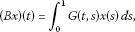(2.1)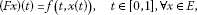(2.2)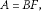(2.3)

where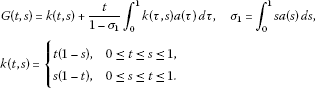It is obvious that the fixed points of the operator A defined by (2.3) are the solutions of the boundary value problem (1.1) (see [15, 16]).

Lemma 2.3 

1. (i)

$B:E\to E$ is a completely continuous linear operator;

2. (ii)

$A:E\to E$ is a completely continuous operator;

3. (iii)

$A=BF$ is quasi-additive on the lattice;

4. (iv)

the eigenvalues of the linear operator B are $\left\{\frac{1}{{\lambda }_{n}},n=1,2,\dots \right\}$ and the algebraic multiplicity of $\frac{1}{{\lambda }_{n}}$ is equal to 1, where ${\lambda }_{n}$ is defined by (H0);

5. (v)

$r\left(B\right)=\frac{1}{{\lambda }_{1}}$, where $r\left(B\right)$ is the spectral radius of the operator B.

## 3 Main results

Let us list some conditions for convenience.

(H1) There exists a constant $b>0$ such that

$f\left(t,x\right)\ge -b,\phantom{\rule{1em}{0ex}}\mathrm{\forall }t\in \left[0,1\right],x\ge 0.$

(H2) There exists a constant $ϵ>0$ such that

where ${\lambda }_{1}$ is defined by (H0).

(H3) $f\left(t,0\right)=0$ uniformly on $t\in \left[0,1\right]$.

(H4)

where ${\lambda }_{n}<\lambda <{\lambda }_{n+1}$, ${\lambda }_{n}$, ${\lambda }_{n+1}$ is defined by (H0).

Theorem 3.1 Suppose that (H0), (H1), (H2), (H3), (H4) are satisfied and n is an odd number in (H4). Then the boundary value problem (1.1) has at least a nontrivial solution.

Proof Choose $0<\delta <ϵ$, then $h={\lambda }_{1}-ϵ+\delta <{\lambda }_{1}$. By (H2), there exists a constant $M>0$ such that

$\frac{f\left(t,x\right)}{x}\le h,\phantom{\rule{1em}{0ex}}\mathrm{\forall }t\in \left[0,1\right],|x|\ge M.$
(3.1)

So, by (3.1) and (H1), we know that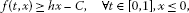(3.2)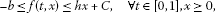(3.3)

where $C={sup}_{0\le t\le 1,|x|.

By (3.2) and (3.3), we have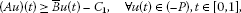(3.4)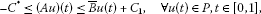(3.5)

where ${C}_{1}=C{\int }_{0}^{1}G\left(t,s\right)\phantom{\rule{0.2em}{0ex}}ds$, ${C}^{\ast }=b{\int }_{0}^{1}G\left(t,s\right)\phantom{\rule{0.2em}{0ex}}ds$, $t\in \left[0,1\right]$, $\overline{B}=hB$, B is defined by (2.1). Obviously, ${C}_{1}\in P$, ${C}^{\ast }\in P$, $\overline{B}:P\to P$ is a positive completely continuous operator. By Lemma 2.3, we have $r\left(B\right)=\frac{1}{{\lambda }_{1}}$, so $r\left(\overline{B}\right)=hr\left(B\right)<{\lambda }_{1}r\left(B\right)=1$.

By (H3), we have $A\theta =\theta$, and

$\left({A}_{\theta }^{\prime }u\right)\left(t\right)={\int }_{0}^{1}G\left(t,s\right){f}_{x}^{\prime }\left(s,0\right)u\left(s\right)\phantom{\rule{0.2em}{0ex}}ds=\lambda {\int }_{0}^{1}G\left(t,s\right)u\left(s\right)\phantom{\rule{0.2em}{0ex}}ds,$
(3.6)

i.e., ${A}_{\theta }^{\prime }=\lambda B$. By Lemma 2.3, 1 is not an eigenvalue of the linear operator ${A}_{\theta }^{\prime }$. Since ${\lambda }_{n}<\lambda <{\lambda }_{n+1}$, n is an odd number, the sum of the algebraic multiplicities for all eigenvalues of ${A}_{\theta }^{\prime }$ lying in $\left(1,+\mathrm{\infty }\right)$ is an odd number. By Lemma 2.1, the operator A has at least one nonzero fixed point. So, the boundary value problem (1.1) has at least one nontrivial solution. □

Theorem 3.2 Suppose (H0), (H2), (H3), (H4) are satisfied and n is an even number in (H4). In addition, assume that

$f\left(t,x\right)x>0,\phantom{\rule{1em}{0ex}}\mathrm{\forall }t\in \left[0,1\right],x\in \left(-\mathrm{\infty },+\mathrm{\infty }\right),x\ne 0.$
(3.7)

Then the boundary value problem (1.1) has at least three nontrivial solutions: one positive solution, one negative solution and one sign-changing solution.

Proof By (3.7), we have

$f\left(t,x\right)>0,\phantom{\rule{1em}{0ex}}\mathrm{\forall }t\in \left[0,1\right],\mathrm{\forall }x>0;\phantom{\rule{2em}{0ex}}f\left(t,x\right)<0,\phantom{\rule{1em}{0ex}}\mathrm{\forall }t\in \left[0,1\right],\mathrm{\forall }x<0.$
(3.8)

By (3.1) and (3.8), (3.4) and (3.5) hold. From (H3), (3.6) holds, and by Lemma 2.3, 1 is not an eigenvalue of the linear operator ${A}_{\theta }^{\prime }$. Since ${\lambda }_{n}<\lambda <{\lambda }_{n+1}$, n is an even number, the sum of the algebraic multiplicities for all eigenvalues of ${A}_{\theta }^{\prime }$ lying in $\left(1,+\mathrm{\infty }\right)$ is an even number.

Obviously, from (3.8) and (2.2), we easily get

$F\left(P\mathrm{\setminus }\left\{\theta \right\}\right)\subset P\mathrm{\setminus }\left\{\theta \right\},\phantom{\rule{2em}{0ex}}F\left(\left(-P\right)\mathrm{\setminus }\left\{\theta \right\}\right)\subset \left(-P\right)\mathrm{\setminus }\left\{\theta \right\}.$
(3.9)

From (2.1), we easily know that $B\left(P\mathrm{\setminus }\left\{\theta \right\}\right)\subset P\mathrm{\setminus }\left\{\theta \right\}$, $B\left(\left(-P\right)\mathrm{\setminus }\left\{\theta \right\}\right)\subset \left(-P\right)\mathrm{\setminus }\left\{\theta \right\}$.

So, by (3.9), we have

$A\left(P\mathrm{\setminus }\left\{\theta \right\}\right)\subset intP,\phantom{\rule{2em}{0ex}}A\left(\left(-P\right)\mathrm{\setminus }\left\{\theta \right\}\right)\subset int\left(-P\right).$

By Lemma 2.2, the boundary value problem (1.1) has at least three nontrivial solutions containing a positive solution, a negative solution and a sign-changing solution. □

Remark By Theorem 3.1 and Theorem 3.2, we can see that the methods used in this paper are different from , and the results are different from .

Example 3.1 We consider the following integral boundary value problem:

$\left\{\begin{array}{c}-{x}^{″}\left(t\right)=f\left(t,x\left(t\right)\right),\phantom{\rule{1em}{0ex}}0\le t\le 1,\hfill \\ x\left(0\right)=0,\phantom{\rule{2em}{0ex}}x\left(1\right)={\int }_{0}^{1}sx\left(s\right)\phantom{\rule{0.2em}{0ex}}ds,\hfill \end{array}$
(3.10)

where

$f\left(t,x\right)=\left\{\begin{array}{cc}5x+t\sqrt{x}-40,\hfill & t\in \left[0,1\right],x\in \left(4,+\mathrm{\infty }\right),\hfill \\ \frac{t-31}{3}\left(x-1\right)+11+t,\hfill & t\in \left[0,1\right],x\in \left(1,4\right],\hfill \\ 10x+\left(1+t\right){x}^{\frac{5}{3}},\hfill & t\in \left[0,1\right],x\in \left[-1,1\right],\hfill \\ \frac{t+29}{7}\left(x+1\right)-11-t,\hfill & t\in \left[0,1\right],x\in \left(-8,-1\right],\hfill \\ 5x+t\sqrt{x},\hfill & t\in \left[0,1\right],x\in \left(-\mathrm{\infty },-8\right].\hfill \end{array}$

By simple calculations, we get that ${\lambda }_{1}\approx 7.53$, ${\lambda }_{2}\approx 37.41$, ${\lambda }_{3}\approx 86.80$, $\lambda =10$. So, ${\lambda }_{1}<\lambda <{\lambda }_{2}$. It is easy to know that the nonlinear term f satisfies (H1), (H2), (H3), (H4). Thus, the boundary value problem (3.10) has at least a nontrivial solution by Theorem 3.1.

Example 3.2 We consider the following integral boundary value problem:

$\left\{\begin{array}{c}-{x}^{″}\left(t\right)=f\left(t,x\left(t\right)\right),\phantom{\rule{1em}{0ex}}0\le t\le 1,\hfill \\ x\left(0\right)=0,\phantom{\rule{2em}{0ex}}x\left(1\right)={\int }_{0}^{1}sx\left(s\right)\phantom{\rule{0.2em}{0ex}}ds,\hfill \end{array}$
(3.11)

where

$f\left(t,x\right)=\left\{\begin{array}{cc}6x+t\sqrt{x},\hfill & t\in \left[0,1\right],x\in \left(4,+\mathrm{\infty }\right),\hfill \\ \frac{t-18}{3}\left(x-1\right)+41+t,\hfill & t\in \left[0,1\right],x\in \left(1,4\right],\hfill \\ 40x+\left(1+t\right){x}^{\frac{5}{3}},\hfill & t\in \left[0,1\right],x\in \left[-1,1\right],\hfill \\ \frac{t+7}{7}\left(x+1\right)-41-t,\hfill & t\in \left[0,1\right],x\in \left(-8,-1\right],\hfill \\ 6x+t\sqrt{x},\hfill & t\in \left[0,1\right],x\in \left(-\mathrm{\infty },-8\right].\hfill \end{array}$

By simple calculations, we get that ${\lambda }_{1}\approx 7.53$, ${\lambda }_{2}\approx 37.41$, ${\lambda }_{3}\approx 86.80$, $\lambda =40$. So ${\lambda }_{2}<\lambda <{\lambda }_{3}$. It is easy to know that the nonlinear term f satisfies (H2), (H3), (H4) and $f\left(t,x\right)x>0$, $\mathrm{\forall }t\in \left[0,1\right]$, $x\ne 0$. The boundary value problem (3.11) has at least three nontrivial solutions containing a positive solution, a negative solution and a sign-changing solution by Theorem 3.2.

## References

1. Gupta CP: Solvability of a three-point nonlinear boundary value problem for a second order ordinary differential equations. J. Math. Anal. Appl. 1992, 168: 540-551. 10.1016/0022-247X(92)90179-H

2. Zhang GW, Sun JX: Multiple positive solutions of singular second order three-point boundary value problems. J. Math. Anal. Appl. 2006, 317: 442-447. 10.1016/j.jmaa.2005.08.020

3. Zhang GW, Sun JX: Positive solutions of m -point boundary value problems. J. Math. Anal. Appl. 2004, 291: 406-418. 10.1016/j.jmaa.2003.11.034

4. Xu X, Sun JX: On sign-changing solution for some three-point boundary value problems. Nonlinear Anal. 2004, 59: 491-505.

5. Ma RY: Nodal solutions for a second-order m -point boundary value problem. Czechoslov. Math. J. 2006, 56: 1243-1263. 10.1007/s10587-006-0092-7

6. Zhang KM, Xie XJ: Existence of sign-changing solutions for some asymptotically linear three-point boundary value problems. Nonlinear Anal. 2009, 70: 2796-2805. 10.1016/j.na.2008.04.004

7. Gallardo JM: Second order differential operators with integral boundary conditions and generation of semigroups. Rocky Mt. J. Math. 2000, 30: 1265-1292. 10.1216/rmjm/1021477351

8. Timoshenko S: Theory of Elastice Stability. McGraw-Hill, New York; 1961.

9. Webb JRL, Infante G: Positive solutions of nonlocal boundary value problems: a unified approach. J. Lond. Math. Soc. 2006, 74: 673-693. 10.1112/S0024610706023179

10. Karakostas GL, Tsamatos PC: Multiple positive solutions of some Fredholm integral equations arisen from nonlocal boundary-value problems. Electron. J. Differ. Equ. 2002, 30: 1-17.

11. Yang ZL: Existence of nontrivial solutions for a nonlinear Sturm-Liouville problem with integral boundary value conditions. Nonlinear Anal. 2008, 68: 216-225. 10.1016/j.na.2006.10.044

12. Jankowski T: Differential equations with integral boundary conditions. J. Comput. Appl. Math. 2002, 147: 1-8. 10.1016/S0377-0427(02)00371-0

13. Yang ZL: Positive solutions of a second order integral boundary value problem. J. Math. Anal. Appl. 2006, 321: 751-765. 10.1016/j.jmaa.2005.09.002

14. Li Y, Li F: Sign-changing solutions for second-order integral boundary value problems. Nonlinear Anal. 2008, 69: 1179-1187. 10.1016/j.na.2007.06.024

15. Zhang XQ, Sun JX: On multiple sign-changing solutions for some second-order integral boundary value problems. Electron. J. Qual. Theory Differ. Equ. 2010, 44: 1-15.

16. Li HT, Liu YS: On sign-changing solutions for a second-order integral boundary value problem. Comput. Math. Appl. 2011, 62: 651-656. 10.1016/j.camwa.2011.05.046

17. Zhang XM, Feng MQ, Ge WG: Existence of solutions of boundary value problems with integral boundary conditions for second-order impulsive integro-differential equations in Banach spaces. J. Comput. Appl. Math. 2010, 233: 1915-1926. 10.1016/j.cam.2009.07.060

18. Feng MQ, Zhang XM, Ge WG: New existence results for higher-order nonlinear fractional differential equation with integral boundary conditions. Bound. Value Probl. 2011., 2011: Article ID 720702

19. Feng MQ, Ji DH, Ge WG: Positive solutions for a class of boundary value problem with integral boundary conditions in Banach spaces. J. Comput. Appl. Math. 2008, 222: 351-363. 10.1016/j.cam.2007.11.003

20. Feng MQ: Existence of symmetric positive solutions for a boundary value problem with integral boundary conditions. Appl. Math. Lett. 2011, 24: 1419-1427. 10.1016/j.aml.2011.03.023

21. Feng MQ, Pang HH: A class of three-point boundary-value problems for second-order impulsive integro-differential equations in Banach spaces. Nonlinear Anal. 2009, 70: 64-82. 10.1016/j.na.2007.11.033

22. Sun JX: Nonlinear Functional Analysis and Applications. Science Press, Beijing; 2008.

23. Sun JX, Liu XY: Computation of topological degree for nonlinear operators and applications. Nonlinear Anal. 2008, 69: 4121-4130. 10.1016/j.na.2007.10.042

24. Sun JX, Liu XY: Computation of topological degree in ordered Banach spaces with lattice structure and its application to superlinear differential equations. J. Math. Anal. Appl. 2008, 348: 927-937. 10.1016/j.jmaa.2008.05.023

25. Liu XY, Sun JX: Computation of topological degree of unilaterally asymptotically linear operators and its applications. Nonlinear Anal. 2009, 71: 96-106. 10.1016/j.na.2008.10.032

## Acknowledgements

The authors would like to thank the reviewers for carefully reading this article and making valuable comments and suggestions. The project is supported by the National Natural Science Foundation of P.R. China (10971179), Research Award Fund for Outstanding Young Scientists of Shandong Province (BS2012SF022, BS2010SF023), Natural Science Foundation of Shandong Province (ZR2010AM035) and SDUST CISE Research Fund.

## Author information

Authors

### Corresponding author

Correspondence to Hongyu Li.

### Competing interests

The authors declare that they have no competing interests.

### Authors’ contributions

All authors typed, read and approved the final manuscript.

## Rights and permissions

Reprints and Permissions

Li, H., Sun, F. Existence of solutions for integral boundary value problems of second-order ordinary differential equations. Bound Value Probl 2012, 147 (2012). https://doi.org/10.1186/1687-2770-2012-147

• Accepted:

• Published:

• DOI: https://doi.org/10.1186/1687-2770-2012-147

### Keywords

• lattice
• fixed point
• integral boundary value problem
• sign-changing solution